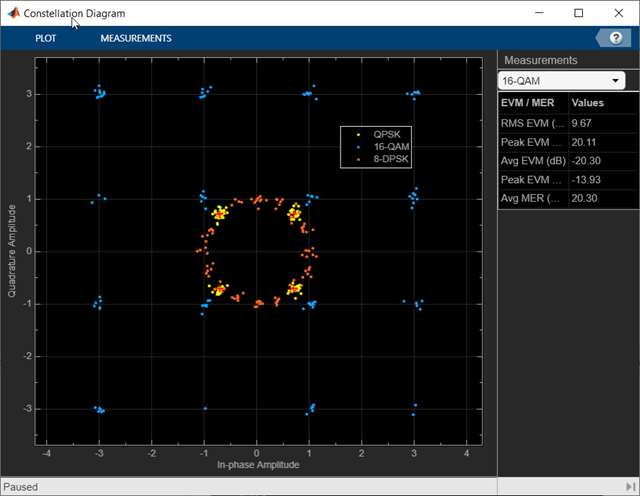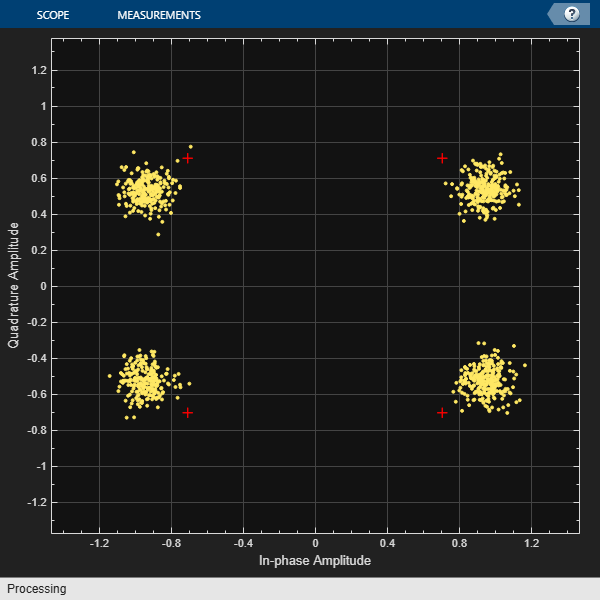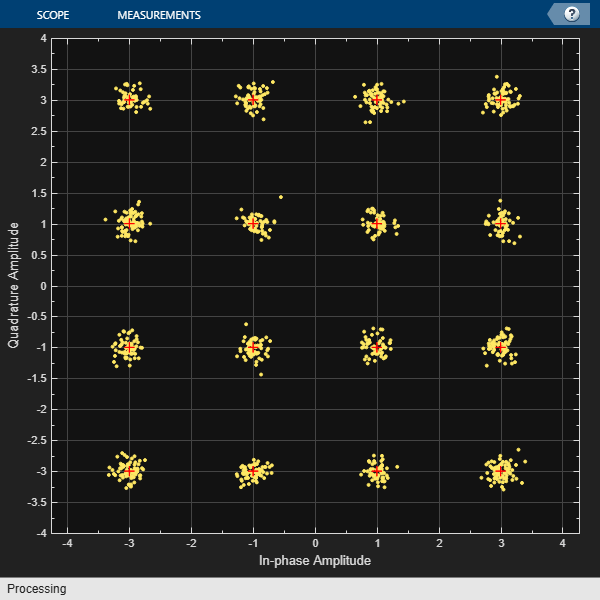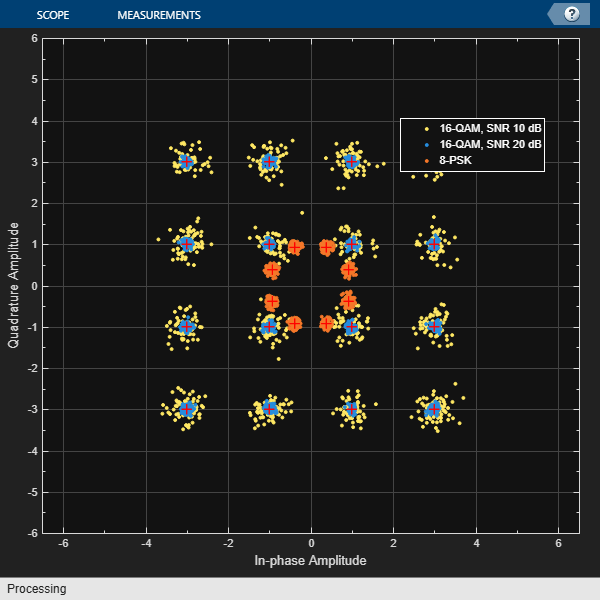comm.ConstellationDiagram

Display constellation diagram for input signals

Description

The comm.ConstellationDiagram System object™ displays real- and complex-valued floating- and fixed-point signals in the IQ plane. Use this System object to perform qualitative and quantitative analysis on modulated single-carrier signals.In the Constellation Diagram window, you can:

• Input and plot multiple signals on a single constellation diagram. To define a reference constellation for each input signal, use the ReferenceConstellation property.

• Select signals in the legend to toggle visibility of individual channels. To display the legend, use the ShowLegend property. For a multichannel signal, specify the input as a matrix with individual signals defined in the columns of the matrix.

• Display calculated error vector magnitude (EVM) and modulation error ratio (MER) measurements for individual signals. To view and configure the measurements, select on the Measurements tab. When multiple signals are input, you can select which signal to use for measurements in the Channel section.

To display constellation diagrams for input signals:

1. Create the comm.ConstellationDiagram object and set its properties.

2. Call the object with arguments, as if it were a function.

Creation

Description

example

constdiag = comm.ConstellationDiagram returns a constellation diagram System object that displays real- and complex-valued floating- and fixed-point signals in the IQ plane.

example

constdiag = comm.ConstellationDiagram(Name,Value) sets properties using one or more name-value arguments. For example, 'SamplesPerSymbol',10 specifies 10 samples for each plotted symbol.

Properties

expand all

Unless otherwise indicated, properties are nontunable, which means you cannot change their values after calling the object. Objects lock when you call them, and the release function unlocks them.

If a property is tunable, you can change its value at any time.

Title of the Constellation Diagram window, specified as a character vector or string scalar.

Tunable: Yes

Data Types: char | string

Number of samples used to represent each symbol, specified as a positive integer. The signal is downsampled by the value of this property before it is plotted.

Tunable: Yes

Data Types: double

Number of samples to skip before plotting points, specified as a nonnegative integer less than the SamplesPerSymbol property value. This value specifies the number of samples to skip when SamplesPerSymbol is greater than 1.

Tunable: Yes

Data Types: double

Source of symbols to display, specified as one of these values.

• 'Input frame length' — The number of symbols to display is equal to the input frame length divided by the SamplesPerSymbol property value.

• 'Property' — The SymbolsToDisplay property specifies the maximum number of symbols to display.

Tunable: Yes

Data Types: char | string

Maximum number of symbols to display, specified as a positive integer. Use this property to limit the maximum number of symbols that the constellation diagram displays when you input long signals. The object plots the most recently received symbols.

Tunable: Yes

Dependencies

To enable this property, set SymbolsToDisplaySource to 'Property'.

Data Types: double

Reference constellations for the input signals, specified as a row vector or cell array of vectors defining the ideal constellation points for each input signal. Input signals can be single channel or multichannel. You can define one reference constellation for each input signal.

• When you specify a row vector, the values apply for all input signals.

• When you specify a cell array, you can specify individual reference constellations for each input signal.

The EVM and MER measurements use the specified reference constellation to calculate the signal quality of the modulated input signal. For more information about the signal quality measurements, see EVM and MER Measurements.

Tunable: Yes

Data Types: double
Complex Number Support: Yes

Marker for the reference constellation display, specified as one of the values listed in this table.

MarkerDescriptionResulting Marker
'o'Circle'+'Plus sign'*'Asterisk'.'Point'x'Cross'_'Horizontal line'|'Vertical line's'Square'd'Diamond'^'Upward-pointing triangle'v'Downward-pointing triangle'>'Right-pointing triangle'<'Left-pointing triangle'p'Pentagram'h'Hexagram'none'No markersNot applicable

Tunable: Yes

Color for reference display constellation, specified as a three-element row vector indicating RGB component colors or as a cell array containing RGB component colors for each input signal.

Tunable: Yes

Data Types: double

Option to display the reference constellation, specified as a logical 1 (true) or 0 (false).

Tunable: Yes

Data Types: logical

Scope window position and size in pixels, specified as a four-element row vector of the form [left bottom width height]. The first two elements in the vector indicate the location of the lower-left corner, and the second elements two specify the size of the window. The default value for the location depends on the screen resolution.

Tunable: Yes

Data Types: double

Option to show grid on the constellation diagram, specified as a logical 1 (true) or 0 (false).

Tunable: Yes

Data Types: logical

Number of input signals, specified as an integer in the range [1, 20]. Each input signal, whether it is a multichannel signal or a single channel signal, becomes a separate channel in the scope.

The total number of channels cannot exceed 20. When you specify multichannel input signals, the maximum number of input signals is limited by the total number of input channels that you define.

When you call the object, the number of inputs that you specify must equal the value of this property.

Data Types: double

Option to display the legend, specified as a logical 0 (false) or 1 (true). The names listed in the legend are the signal names specified by the ChannelNames property. The legend does not display until you call the object with an input signal.

In the scope legend, click a signal name to toggle the signal visibility in the scope.

Tunable: Yes

Data Types: logical

Names for the input channels, specified as a cell array of strings or character vectors. If you do not specify names, the object labels the channels as Channel 1, Channel 2, etc.

These names appear in the legend, the Measurements tab, and the Measurements Setting pane.

Example: {'8-QAM','8-PSK'} specifies the names for two input channels to 8-QAM and 8-PSK.

Tunable: Yes

Data Types: cell

Option to plot the signal trajectory, specified as a logical 0 (false) or 1 (true). Setting this property to true displays the trajectory between constellation points for the plotted signals. To view the signal trajectory, select on the Plot tab.

Tunable: Yes

Data Types: logical

Option to add color fading effect, specified as a logical 0 (false) or 1 (true). When you set this property to true, the points in the display fade as the interval of time after they are first plotted increases. This animation resembles an oscilloscope display.

Tunable: Yes

Data Types: logical

Plot title, specified as a character vector or string scalar.

Tunable: Yes

Data Types: char | string

x-axis limits, specified as a two-element row vector of the form [xmin xmax]. The first element is the minimum x-axis value, and the second element is the maximum x-axis value.

Tunable: Yes

Data Types: double

y-axis limits, specified as a two-element row vector of the form [ymin ymax]. The first element is the minimum y-axis value, and the second element is the maximum y-axis value.

Tunable: Yes

Data Types: double

x-axis label, specified as a character vector or string scalar.

Tunable: Yes

Data Types: char | string

y-axis label, specified as a character vector or string scalar.

Tunable: Yes

Data Types: char | string

Option to calculate and display the EVM and MER measurements, specified as a logical 0 (false) or 1 (true).

Tunable: Yes

Data Types: logical

Window length for the EVM and MER measurements, specified as 'Current display', 'All displays', or an integer in the range [2, SymbolsToDisplay].

Tunable: Yes

Data Types: char | string | double

EVM normalization method, specified as 'Average constellation power' or 'Peak constellation power'. For more information, see EVM and MER Measurements.

Tunable: Yes

Usage

Description

example

constdiag(signal1, ..., signalN) displays up to N signals in one constellation diagram, where N is the NumInputPorts property value.

Input Arguments

expand all

Signals, specified as separate arguments of Nsym-by-1 column vectors or Nsym-by-Nchannel matrices. Nsym is the number of symbols, and Nchannel is the number of input signal channels. Signals can have different data types and dimensions.

You must specify N input arguments, where N is the NumInputPorts property value. You can visualize up to 20 individual or collective signal channels in the constellation diagram. For example, if you create a two-channel signal for every input, then you can define up to 10 input arguments.

Example: [sig1_1,sig1_2],sig2 specifies two signals, provided that sig1_1, sig1_2, and sig2 are single channel column vector signals. the first, [sig1_1,sig1_2], specifies a two-channel signal (constructed by concatenating two column vectors into a matrix). The second signal, sig2, specifies a single channel.

Data Types: double
Complex Number Support: Yes

Object Functions

To use an object function, specify the System object as the first input argument. For example, to release system resources of a System object named obj, use this syntax:

release(obj)

expand all

 show Show scope window hide Hide scope window isVisible Determine visibility of scope window
 step Run System object algorithm release Release resources and allow changes to System object property values and input characteristics reset Reset internal states of System object

Examples

collapse all

QPSK-modulate random data symbols and apply an amplitude imbalance to the signal. Pass the signal through a noisy channel. Display the resultant constellation.

Create a constellation diagram System object. Because the default reference constellation for the object is QPSK, setting additional properties is not necessary.

constDiagram = comm.ConstellationDiagram;

Generate random data symbols, and then apply QPSK modulation.

data = randi([0 3],1000,1);
modData = pskmod(data,4,pi/4);

Apply an amplitude imbalance to the modulated signal.

txSig = iqimbal(modData,5);

Pass the transmitted signal through an AWGN channel, and then display the constellation diagram. The data points shift from their ideal locations.

rxSig = awgn(txSig,20);
constDiagram(rxSig)Apply 16-QAM modulation, transmit data using an AWGN channel, and display the signal constellation.

Create a 16-QAM reference constellation.

M = 16;
refC = qammod(0:M-1,M);

Create a constellation diagram System object, specifying the constellation reference points and axes limits.

constDiagram = comm.ConstellationDiagram('ReferenceConstellation',refC, ...
'XLimits',[-4 4],'YLimits',[-4 4]);

Generate random 16-ary data symbols.

data = randi([0 M-1],1000,1);

Apply 16-QAM modulation.

sym = qammod(data,M);

Pass the modulated signal through an AWGN channel.

rcv = awgn(sym,15);

Display the constellation diagram.

constDiagram(rcv)Display the constellation of multi-input and multichannel modulated signals. Plot a multichannel signal with two 16-QAM signals for the first input and one 8-PSK signal for the second input.

Create a 16-QAM and an 8-PSK reference constellation.

M = 16;
refQAM = qammod(0:M-1,M);
S = 8;
refPSK = pskmod(0:S-1,S,pi/8);

Create a constellation diagram System object, specifying reference constellations for the two input signals. The object applies a single reference constellation for all the channels of an individual multichannel signal input, but separate input signals can specify separate reference constellations.

constDiag = comm.ConstellationDiagram(2, ...
'ReferenceConstellation',{refQAM,refPSK}, ...
'ShowLegend',true, ...
'XLimits',[-6 6],'YLimits',[-6 6], ...
'ChannelNames', ...
{'16-QAM, SNR 10 dB','16-QAM, SNR 20 dB','8-PSK'});

Generate random data symbols, modulate the symbols, and add AWGN with two different SNRs to yield two received signals. Use SNR values of 10 and 20 dB.

d = randi([0 M-1],1000,1);
dQAM = qammod(d,M);
rcv1_1 = awgn(dQAM,10);
rcv1_2 = awgn(dQAM,20);
d = randi([0 S-1],1000,1);
dPSK = pskmod(d,S,pi/8);
rcv2 = awgn(dPSK,20);

For the first input, create a multichannel signal by concatenating the two received 16-QAM signals. For the second input uses a single channel 8-PSK signal.

Display the constellation diagram of the multi-input and multichannel signals.

constDiag([rcv1_1,rcv1_2],rcv2);expand all

Tips

• To capture a simple signal constellation snapshot, use the scatterplot function.

• To calculate signal quality, show signal trajectory, capture constellations for multiple signals, or maintain state between calls, use a comm.ConstellationDiagram System object.